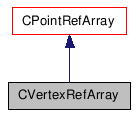Public Member Functions
CVertexRefArray Class Reference

## Detailed Description

Represents an array of Vertex objects.

Warning:
This specialized array is returned by PolygonMesh::GetVertices, PolygonFace::GetVertices and Edge::GetVertices, it is not meant to be created and modified in user-defined functions. If you want to add and remove arbitrary items to a collection, you must use a CRefArray instead.
PolygonMesh::GetVertices, PolygonFace::GetVertices, Edge::GetVertices
Example:
```        using namespace XSI;
Application app;
Model root = app.GetActiveSceneRoot();

X3DObject myGrid;
root.AddGeometry( L"Grid", L"MeshSurface", L"", myGrid );

PolygonMesh mesh(myGrid.GetActivePrimitive().GetGeometry());
CVertexRefArray vertices(mesh.GetVertices());

LONG lCount = vertices.GetCount();
for (LONG i=0; i<lCount; i++ )
{
Vertex vertex(vertices.GetItem(i));

CVertexRefArray neighbors(vertex.GetNeighborVertices(1));
app.LogMessage( L"Number of neighbor vertices for Vertex " +
CValue(i).GetAsText() + L": " +
CValue(neighbors.GetCount()).GetAsText() );
}
```

`#include <xsi_vertex.h>`

Inheritance diagram for CVertexRefArray:[legend]

List of all members.

## Public Member Functions

CVertexRefArray (const CVertexRefArray &in_array)
CRef  GetItem (LONG in_index) const
CRef  GetItem (const CString &in_name) const
LONG  GetCount () const
bool  IsValid () const
CVertexRefArray  GetNeighborVertices (LONG in_lDistance=1) const
CEdgeRefArray  GetNeighborEdges (LONG in_lDistance=1) const
CPolygonFaceRefArray  GetNeighborPolygons (LONG in_lDistance=1) const
CVertexRefArray  GrowNeighborVertices (LONG in_lDistance=1) const
CDoubleArray  GetCreaseArray () const

## Constructor & Destructor Documentation

 CVertexRefArray ( const CVertexRefArray & in_array )

Copy constructor.

Parameters:
 in_array constant CVertexRefArray reference object.

## Member Function Documentation

 CRef GetItem ( LONG in_index ) const `[virtual]`

Returns a Vertex object at a specified index in the array.

Parameters:
 in_index The index of the Vertex.
Returns:
CRef Vertex object.

Reimplemented from CPointRefArray.

 CRef GetItem ( const CString & in_name ) const `[virtual]`

Returns the Vertex object in the array matching the specified name.

Parameters:
 in_name The name of the Vertex.
Returns:
CRef Vertex object.

Reimplemented from CPointRefArray.

 LONG GetCount ( ) const `[virtual]`

Returns the number of Vertex objects in the array.

Returns:
LONG Number of Vertex objects.

Reimplemented from CPointRefArray.

 bool IsValid ( ) const `[virtual]`

Returns true if this array is a valid object or false otherwise.

Returns:
bool True if valid, false otherwise.

Reimplemented from CPointRefArray.

 CVertexRefArray GetNeighborVertices ( LONG in_lDistance = `1` ) const

Returns an array of Vertex neighbors for all vertices contained in this array, within a given distance.

Parameters:
 in_lDistance Value representing the degree of neighborhood
Returns:
CVertexRefArray An array of Vertex objects
 CEdgeRefArray GetNeighborEdges ( LONG in_lDistance = `1` ) const

Returns an array of Edge neighbors for all vertices contained in this array, within a given distance.

Parameters:
 in_lDistance Value representing the degree of neighborhood
Returns:
CEdgeRefArray An array of Edge objects
 CPolygonFaceRefArray GetNeighborPolygons ( LONG in_lDistance = `1` ) const

Returns an array of PolygonFace neighbors for all vertices contained in this array, within a given distance.

Parameters:
 in_lDistance Value representing the degree of neighborhood
Returns:
CPolygonFaceRefArray An array of PolygonFace objects
 CVertexRefArray GrowNeighborVertices ( LONG in_lDistance = `1` ) const

Returns an array of adjacent Vertex neighbors for all vertices contained in this array, within a given distance.

Parameters:
 in_lDistance Value representing the degree of neighborhood
Returns:
CVertexRefArray An array of Vertex objects
 CDoubleArray GetCreaseArray ( ) const

Returns an array of crease values for all vertices contained in this vertex array.

Returns:
CDoubleArray An array of crease value.
Since:
3.5.1

The documentation for this class was generated from the following file: